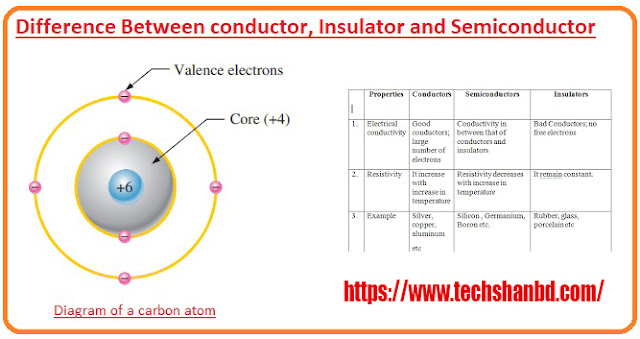Electrical-Engineering-Fundamentals

# Conductive, semiconductor and non-conductiveDifference-Between-conductor-Insulator-and-Semiconductor | https://techshanbd.com/

Question-1. What is a conductor? Or, what is a conductor? Or, what is a conductor? Give some examples.

Answer: All the substances through which current can flow easily are called conductive. Example: Generally, all metallic substances are considered to be conductive. In addition, soil, coal, animal bodies act as conductors.

Question-2. What is an insulator? Or, what is non-conductive? Give some examples. Or, what is an insulator?

Answer: Substances through which current cannot flow easily are called non-conductive. Example: Acts as conductor or insulator of rubber, paper, dry wood, glass, etc.

Question-3. What is a semiconductor? An Or, define a semiconductor with examples. Or, write the definition of semiconductor. Or, define semiconductor. Or, write the definition of semiconductor. Or, define a semiconductor. Or, what is a semiconductor?

Answer: All the substances through which a very small amount of current flows, that is, all the substances carrying current. He calls all substances between the conductive and non-conductive semiconductors.

Question-4. Write the names of the six conductors.

Question-5. What is the range of resistivity of semiconductor materials?

Question-6. What does a regulator of a conductor depend on? Or, in what ways does the regulator of the conductor depend? Or, on what factors does the conductivity of the conductor depend? * Or, on what date does the conductor’s registry depend?

Answer: Resistance depends on the length of the conductor, the area of ​​the cross-section, the material, and the temperature.

Question-7. What is a free electron?

Answer: The electrons of that atom that are lightly bound to the nucleus and which are easily conductive. When they flow through, they are called free electrons.

Question-8. According to electron theory, what is a semiconductor?.

Answer: Resistance depends on the length of the conductor, the area of ​​the cross section, the material and the temperature.

Question-9. What is the unit of relative radiation in the SI system? Or, s. What is the unit of resistivity in the I method?

Question-10. What is the unit of relative radiation in the MKS method?

Question-11. Write the definition of relative rash. Or, what is resistivity? Or, what is the relative rash?; Or, define relative radiance. Or, define relative rash.

Ans: Two opposite surfaces of a cube of one meter or one centimeter or one-inch arm. The intermediate resistance is called the relative resistance of that substance.

Question-12. If the diameter of a conductor increases 2 times, how many times will its current carrying capacity increase?

Answer: As the radius is proportional to the square of the diameter, the radius will decrease four times and the current-carrying capacity will increase four times. Will get.

Question-13. What is the name of the person through whom electricity can easily flow?

Question-14. What is the unit of relative radiation in the CGS system?

Question-15. Does the resistance of the insulator vary with increasing heat?

Question-16. What happens to the radius when the diameter of the conductor is reduced by half?

Question-17. What do you mean by relative conductance?

Answer: Conductors of two opposite surfaces of a cube 1 m long, 1 m wide, and 1 m high are called relative ‘* conductors’.

Question-18. What is the subject of the conductor’s radiation?

Answer: Depending on the length, cross-section, material, and temperature of the conductor.

Question-19. Write the units of relative radius. [Or, write the unit of relative rash.

Answer: M. K. S method 2m .. C.G.S Foco 12-cm. F.P.S foco 12-inch 71 12-ft.

Question-20. In the case of Radha, what will be the change in the value of A?

Answer: The resistance (R) of the conductor will decrease.

Question-21. What are conductors and insulators?

Answer: Conductors: Substances through which electricity can easily pass are called conductors. Examples: Copper, Aluminum, Leah, Saina, and Silver. . Insulators: Materials that cannot conduct electricity at all are called insulators. * Says. Such as glass, porcelain, rubber, and paper.

Question-22. Write the names of the six non-existent.

Answer: porcelain, rubber, plastic, ebonite, mica, dry wood.

Question-23. Write the names of the six semiconductors?

Answer: Silicon, Germanium, Selenium, Carbon, Arsenic, Antimony.

Question-24. What is a valence electron?

Answer: The electrons in the last orbit of an atom are called valence electrons.

Question-25. What is a closed electron?

Answer: All the electrons in an atom that have a strong bond with the nucleus are called closed electrons.

Question-26. According to electron theory, what is a conductor?

Answer: According to the electron theory, the number of electrons in the outer chamber of all matter is less than 4, They are called conductors.

Question-27. According to electron theory, what is non-conductive?

Answer: Matter that has 4 electrons in the outer cell of the atom is called non-conductive.

Question-28. If the length and cross-section of the conductor are known, then the resistor of the conductor can be determined by any formula: Is done?